American Journal of Modern Physics
Volume 5, Issue 2-1, March 2016, Pages: 46-55

Studies on Santilli Three-Body Model of the Deuteron According to Hadronic Mechanicsi

Sudhakar S. Dhondge

Department of Chemistry, S. K. Porwal College, Nagpur, India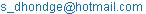Sudhakar S. Dhondge. Studies on Santilli Three-Body Model of the Deuteron According to Hadronic Mechanics. American Journal of Modern Physics. Special Issue: Issue II: Foundations of Hadronic Mechanics. Vol. 5, No. 2-1, 2016, pp. 46-55. doi: 10.11648/j.ajmp.2016050201.14

Received: July 8, 2015; Accepted: July 9, 2015; Published: May 18, 2016

Abstract: In this paper, we outline the inapplicability (rather than the violation) of quantum mechanics for the representation of the synthesis of the neutron from the Hydrogen atom in the core of a star, and we outline the corresponding inability of quantum mechanics for a consistent representation of all characteristics of the deuteron as a two-body state of one proton and one neutron in its ground state. We then outline the first representation of all characteristics of the neutron achieved by R. M. Santilli via a a generalized two-body bound state of one proton and one electron in conditions of total mutual penetration according to the laws of hadronic mechanics, thus implying the mutation of particles into isoparticles under the Lorentz-Santilli isosymmetry. We then outline the first representation of all characteristics of the deuteron also achieved by R. M. Santilli via a generalized three-body bound state of two isoprotons and one isoelectron, including the first known exact and time invariant representation of the deuteron spin, magnetic moment, binding energy, stability, charge radius, dipole moment, etc. We finally study further advances of Santilli three-body model of the deuteron in preparation of its extension to all nuclei, such as: the admission of exact analytic solution for the structure of the deuteron as a restricted three-body system; the validity in first approximation of the structure of the deuteron as a two-body system of one isoproton and one iso neutron; the importance for the representation of experimental data of the deformability of the charge distribution of the proton and the neutron which is prohibited by quantum mechanics but readily permitted by hadronic mechanics in the notion of isoparticle; and other aspects.

1. Introduction

The nucleus of deuterium is called a deuteron and it contains one proton and one neutron, whereas the far more common hydrogen nucleus contains no neutron. The isotope name is formed from the Greek deuterons meaning "second", to denote the two particles composing the nucleus. Thus Deuteron is normally considered as the combination of proton and neutron and thus it is considered as a two body system by quantum mechanical bound state. It is the simplest bound state of nucleons and therefore gives us an ideal system for studying the nucleon-nucleon interaction. In analogy with the ground state of the hydrogen atom, it is reasonable to assume that the ground state of the deuteron also has zero orbital angular momentum L = 0. However the measured total angular momentum is J = 1 (one unit of h/2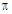) thus it obviously follows that the proton and neutron spins are parallel:. On the other hand, its high stability is to the tune of 2.2 MeV. The stability of deuteron plays a very important part of the existence of the universe.

The structure of deuteron and its physical properties were first proposed by Santilli [1,2]. Although Deuteron is a simple molecule, quantum mechanics has been unable to explain its different properties like the spin, magnetic moment, binding energy, stability, charge radius, dipole moment, etc. The magnetic moment of deuteron was for the first time represented exactly by Santilli . Also for the first time the notion of isoproton and isoelectron was introduced by Santilli [4,5], which was further elaborated by him [6,7]. He made Rutherford’s conjecture of neutron a quantitative description based on his Hadronic Mechanics [8-10]. Santilli under the covering laws of Hadronic Mechanics has demonstrated and established that all nuclei and therefore all the matter at large are supposed to be composed of protons and electrons in their isoprotons and isoelectrons realization characterized by Lorentz-Santilli isosymmetry [4,5,8]. The conception of nuclei as quantum mechanical bound states of proton and neutron remains valid but only as a first approximation. Thus, Santilli’s reduction of the neutron to a hadronic bound state of a proton and an electron suggests the reduction of all nuclei and, therefore, all matter in the universe, to protons and electrons. However, on technical grounds, the constituents of nuclei are given by protons and electron in their form mutated by contact non-Hamiltonian, thus nonunitary interactions called isoprotons and iso- electrons [5,11] (for further details see [6,7] and technically defined as isounitary irreducible representations of the Lorentz-Poincare-Santilli isosymmetry.

Hadronic mechanics not only allows the reduction of a nuclei into (iso) protons and (iso) electrons, but also achieves, for the first time, a numerically exact and invariant representation of various nuclear data beyond any dream of representation via quantum mechanics.

For the sake of some sort of continuity we start in the next Section with a very brief description of neutron structure based on Santilli hadronic mechanics and then would devote all succeeding Sections to hadronic mechanics of deuteron as developed by Santilli.

2. A Brief Review of Neutron Structure Based on Santilli’s Hadronic Mechanics

In the history of science Santilli for the first time quantified the Rutherford conjecture that a neutron is indeed a compressed hydrogen atom using his hadronic mechanics. The main motivation to develop corresponding hadronic mechanics has been the inadequacy of quantum mechanics to arrive at experimentally established properties of neutron e.g. its spin, magnetic moment, its stability within nucleus (an isolated neutron is unstable having half life of about 10 min), etc. For the details of all these aspects can be found in [8-10]. However, herein we recall only the main features of Santilli’s quantification of neutron structure and synthesis to illustrate the continuity of nuclear structure from neutron to deuteron according to hadronic mechanics.

In order to make Rutherford’s conjecture a quantitative one he proposed a model in which the wave packets of an electron and a proton mutually overlap to form a dynamic union such that electron revolves around proton as shown in Figure 1.

In other words, the proton and the electron are actual physical constituents of the neutron in our space-time, not in their conventional quantum mechanical states, but in generalized states due to the total penetration of the wave packet of the electron within the hyperdense proton, for which Santilli has suggested the names of "isoproton, "here denoted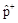, and "isoelectron," here denoted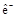, these new states are technically realized as irreducible isorepresentation of the Lorentz-Poincaré-Santilli isosymmetry. In this way he studied the representation of "Rutherford’s compression" of the Hydrogen atom into a neutron inside a star via a non-unitary transform of the conventional structure of the Hydrogen atom (HA).Figure 1. A conceptual view of Rutherford’s compression of the electron inside the hyperdense proton in singlet coupling (necessary for stability), resulting in the constrained orbital angular momentum of the electron under which the total angular momentum of the electron is zero and the spin of the neutron coincides with that of proton.

Thus the mutated electron and proton as shown in Figure 1 are termed as isoelectron and isoproton respectively. The iso-prefix stems from the need of Santilli isomathematics  to describe the process of the said mutation. The said mutation gets mathematically expressed as,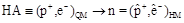(1)

where subscripts QM and HM stands for the horizons of quantum mechanics and hadronic mechanics respectively. From the model of Figure 1 it is evident that the dimensions of interaction between isoelectron and isoproton are of 1 fm or less. But to maintain an electron within such a short nuclear volume very strong attractive force is needed because the conventional electrostatic attraction at such a short distances turns out to be grossly inadequate. This then indicated that an external trigger is operating that forces an electron to penetrate within the hyperdense medium of a proton. This in hadronic mechanics has been quantified through corresponding Hulthén potential, which produces very large attractive force compared to the conventional electrostatic force.

The reader is advised to refer to the references cited herein for the details of the Rutherford-Santilli model of neutron and its synthesis both in Stars and in laboratory.

3. Santilli’s Structured Model of Deuteron as a Hadronic Bound State of Two Protons and One Electron

Santilli considerd deuteron as a hadronic bound state of two protons and one electron verifying the laws and symmetries of hadronic mechanics. According to him:

1.   The deuteron is a stable light, natural isotope that, as such, is reversible over time.

2.   Thus Santilli assumes the quantum mechanical structure less of the deuteron (denoted as "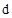")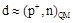(2)

as valid in first approximation, and reduces the deuteron to two protons and one electron according to the structure: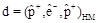(3)

In the above equation all the constituents are isoparticles, namely, two iso- protons and one isoelectron. Their iso-character has been depicted by (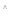) over the symbols.

3.   Contrary to expectations, contact interactions generate a special version of restricted three body system that admits an exact analytic solution.

In this communication we intend to review the insufficiencies of quantum mechanics for a quantitative representation of experiential data on the deuteron and then review their exact and invariant representation via Santilli’s isomechanics and underlying isomathematics.

3.1. Insufficiencies of Quantum Mechanics to Adequately Describe the Structure of Deuteron

3.1.1. Quantum Mechanics has been Unable to Represent or Explain the Stability of the Deuteron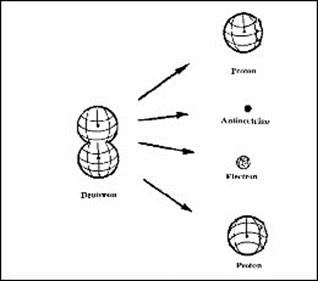Figure 2. Three body model of the deuteron.

This problem might be also due to unavailability of the technical literature of quantitative numerical proofs that, when bonded to a proton, the neutron cannot decay, as an evident condition for stability. Thus the stability of the deuteron has been left fundamentally unexplained by quantum mechanics till date. Santilli illustrated the inability by quantum mechanics to represent the stability of the deuteron, since the neutron is naturally unstable and, therefore, the deuteron should decay into two protons, an electron and the hypothetical antineutrino. Even today, no reason is known that why neutron should become stable when coupled to a proton. Santilli represented three body model of the deuteron and its stability as shown in Figure 2.

3.1.2. Quantum Mechanics has been Unable to Represent the Spin 1 of the Ground State of the Deuteron

According to quantum mechanics the most stable bound state of two particles is with the opposite spins and hence should have SPIN ZERO. No such state has been detected in the deuteron. Thus quantum mechanics has been unable to represent the spin 1 of the ground state of the deuteron. This is illustrated in Figure 3.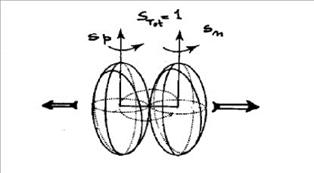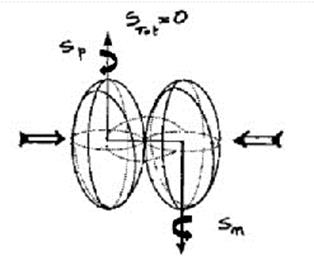Figure 3. Figures above represent the impossibility of quantum mechanics to represent the spin 1 of the deuteron in a way compatible with its size. First figure explains how spin 1 can solely be achieved with a triplet coupling in which case no stable nucleus is conceivable due to very strong repulsive forces at the distance of nuclear forces. Thus only stable state is the singlet but in this case the total angular momentum is zero, in disagreement with experimental evidence.

3.1.3. Quantum Mechanics has been Unable to Reach an exact Representation of the Magnetic Moment of the Deuteron

It has been observed that non-relativistic quantum mechanics misses 0.022 Bohr units corresponding to 2.6% of the experimental value. Relativistic corrections reduce the error down to about 1% but under highly questionable theoretical assumptions, such as the use for ground state of a mixture of different energy levels that are assumed to exist without any emission or absorption of quanta as expected by quantum mechanics. The situation becomes worst for the magnetic moments of heavier nuclei.

3.1.4. Quantum Mechanics has been Unable to Identify the Physical Origin of the Attractive Force that Binds Together the Proton and the Neutron in the Deuteron

Since the neutron is neutral, there is no known electrostatic origin of the attractive force needed for the existence of the deuteron. The only Coulomb force for the proton-neutron system is that of the magnetic moments, which force is REPULSIVE for the case of spin 1 with parallel spin. Therefore, a "strong" force was conjectured and its existence was subsequently proved to be true.

3.1.5. Quantum Mechanics has also been Unable to Treat the Deuteron Space Parity in a Way Consistent with the Rest of the Theory

The experimental value of the space parity of the deuteron is positive for the ground state, because the angular momentum L is null. However, nuclear physicists assume for the calculation of the magnetic moment of deuteron that the ground state is a mixture of the lowest state with L = 0 with other states in which the angular momentum is not null. This produces incompatibility of these calculations with the positive parity of the ground state.

3.2. Inferences

Thus from above discussion we can infer that, after about one century of research, quantum mechanics has left unresolved fundamental problems even for the case of the smallest possible nucleus, the deuteron, with progressively increasing unresolved problems for heavier nuclei. Following these insufficiencies, any additional belief on the final character of quantum mechanics in nuclear physics is a sheer political posture in disrespect of the societal need to search for a more adequate mechanics.

Not only quantum mechanics is not exactly valid in nuclear physics, but the very assumption of neutrons as nuclear constituents is approximately valid since neutrons are composite particles. Therefore, the main objective of this chapter is the identification of stable, massive physical constituents of nuclei and their theoretical treatment that admits in first approximation the proton-neutron model, while permitting deeper advances.

The replacement of protons and neutrons with the hypothetical quark is mathematically significant, with the clarification that, in Santilli’s view, quarks cannot be physical particles because, as stresses several times by Santilli, quarks are purely mathematical representations of a purely mathematical symmetry realized in a purely mathematical internal unitary space without any possible formulation in our spacetime (because of the O’Rafearthaigh’s theorem).

Consequently, quark masses are purely mathematical parameters and cannot be physical inertial masses. As also stressed several times, on true scientific grounds, inertial masses can only be defined as the eigenvalues of the second order Casimir invariant of the Lorentz-Poincaré symmetry. But this basic symmetry is notoriously inapplicable for the representation of quarks because of their particular features. Therefore, quark "masses" cannot have inertia. Additionally, Santilli points out that the hypothetical orbits of the hypothetical quarks are excessively small to allow an exact representation of nuclear magnetic moments via their polarization. In fact, various attempts have been made in representing magnetic moments when reducing nuclei to quarks with the result of bigger deviations from experimental data than those for the proton-neutron structure. Similar increases of the problematic aspects occur for all other insufficiencies of quantum mechanics in nuclear physics. Consequently, the reduction of nuclei to quarks will be ignored hereon because of its excessive deviation from solid physical foundations as well as experimental data.

In conclusion, quarks can indeed be considered as replacements of protons and neutrons, with the understanding that nuclei made up of quarks cannot have any weight, since, according to Albert Einstein, gravity can solely be defined for bodies existing in our spacetime.

It is evident from the above facts that quantum mechanics has been unable to treat the deuteron space parity, in a way consistent with the rest of the theory [1,8,10]. Thus quantum mechanics has not been able to solve fundamental problems even for the case of the smallest possible nucleus, the deuteron, with progressively increasing unresolved problems for heavier nuclei.

4.1. Deuteron Structure

The nuclear force solely applies up to the distance of 10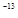cm, which distance coincides with the charge radius of the proton as well as the electron wavepacket, and that the sole stable orbit for the two protons under contact strong interactions is the circle. The size of the deuteron then forces the charge distribution of two protons as essentially being in contact with each other. It can be said that the electron is totally immersed within a proton, expectedly exchanging its penetration from one proton to the other.

Now the spin of the deuteron in its ground state is 1; the spin of the protons is 1/2; the spin of the isoelectron is 1/2; and that the mutated angular momentum of the isoelectron is -1/2. So Santilli assumed the structure of the deuteron as being composed of two un-mutated protons with parallel spins rotating around the central isoelectron to allow the triplet coupling of protons, and then the two coupled particles in line have an orbital motion around the isoelectron at the center, resulting in the first approximation in the following hadronic structure model of the deuteron .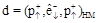(4)

Thus, proton is the only stable particle and neutron is unstable, comprising of proton and electron. Santilli assumed that nuclei are a collection of protons and neutrons, in first approximation, while at a deeper level a collection of mutated protons and electrons. It has been proved that a three-body structure provides the only known consistent representation of all characteristics of the deuteron, first achieved by R. M. Santilli. Thus Coulomb and contact attractive forces in pair-wise singlet couplings proton-isoelectron are so strong to overcome Coulomb repulsion among the two protons and form a bound state that is permanently stable when isolated, as already established for the valence bond and Cooper pairs of identical electrons.

Volodymyr Krasnoholovets has tried to resolve the above anomalies in his recent paper . He analyzed the problem of the deuteron from the viewpoint of the constitution of the real space that he developed. He concluded that the nucleus does not hold the electrons in the orbital position and polarized inertons [14-16] of atomic electrons directly interact with the nucleus. He also analyzed the problem of the motion of nucleons in the deuteron, which takes into account their interaction with the space and concluded that nucleons in the deuteron oscillate along the polar axis and also undergo rotational oscillations. In other words, the nucleons execute radial and rotationally oscillatory motions. Trying to account for the reasons for nuclear forces, he has analyzed major views available in the literature including quantum field theories, hadronic mechanics, and even the Vedic literature.

R. M. Santilli in 1998 provided the consistent representation of all the characteristics of the deuteron using its three body model  that involves isomathematics based methods of hadronic mechanics. His hadronic mechanics method explains the strong attraction between protons and neutrons via the Hulthén potential concept . Thus the hadronic mechanics:

1.   could successfully explain the experimental value of spin 1 of the deuteron;

2.   offered the exact and invariant representation of the total magnetic moment of the deuteron;

3.   provided a physical insight into the deuteron size and charge.

4.2. Size of Deuteron

It has been observed experimentally that the proton has the following values for the charge radius and diameter (size)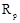= 0.8x 10= 0.8 fm;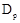= 1.6 fm. Whereas, the value of the size of the deuteron given in literature is: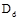= 4.31 fm.

Structure model represented by equation 4 does indeed fully justifies the above data in accordance with Figure 4. In fact, the above data indicate that the charge radii of the two protons are separated by approximately 1.1 fm, namely, an amount that is fully sufficient, on one side, to allow the triplet alignment of the two protons as in the upper part of Figure 4 and, on the other side, to generate contact nonlocal effects from the penetration of the wave packet (here referred to the square of the probability amplitude) of the central spinning electron within the two peripheral protons.Figure 4. Represents the structure of the deuteron as a restricted three body of two un-mutated protons (due to their weight) and one mutated electron. The top view uses the very effective "gear model" to avoid the highly repulsive triplet couplings, while the bottom view is the same as the top view, the particles being represented with overlapping spheres.

4.3. Representation of the Stability of the Deuteron

As indicated earlier, the lack of a quantitative representation of the stability of the deuteron when composed by the stable proton and the unstable neutron has been one of the fundamental problems left unsolved by quantum mechanics in about one century of research.

By comparison, protons and electrons are permanently stable particles. Therefore, structure model equation (4) resolves the problem of the stability of the deuteron in a simple, direct, and visible way. The deuteron has no unstable particle in its structure and, consequently it is stable due to the strength of the nuclear force.

In fact, as shown below, the Coulomb and contact attractive forces in pair-wise singlet couplings proton-isoelectron are so "strong" to overcome Coulomb repulsion among the two protons and form a bound state that is permanently stable when isolated, as already established for the valence bond and Cooper pairs of identical electrons.

4.4. Deuteron Charge

Model given by equation 4 represents the deuteron positive charge +e. This is due to the fact that hadronic mechanics generally implies the mutation of all characteristics of particles, thus including the mutation of conventional charges Q, and so that mutated charge of the deuteron constituents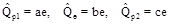(5)

where a, b, c are positive-definite parameters, and e is the elementary charge. These mutations are necessary for consistency with other aspects, such as the reconstruction of the exact isospin symmetry in nuclear physics. However, these mutations are only internal, under the condition of recovering the conventional total charge +e for the system as a whole, as it is the case for closed non-Hamiltonian systems. Consequently, the charge mutations are subject to cancelation in such a way to yield the total charge +e, i.e.,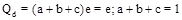(6)

However, the mutations of the charge is expected to be quite small in value as being a second order effect ignorable at a first approximation, the deuteron structure does not require the mutual penetration of the charge distribution of protons.

4.5. Representation of the Deuteron Spin

According to quantum mechanics the most stable state between two particles with spin 1/2 is the singlet, for which the total spin is zero. Thus for the ground state of the deuteron as a bound state of a proton and a neutron should have spin zero. This is exactly contrary to the experimental value of spin 1. When the deuteron is assumed to be a three-body bound state of two protons with an intermediate electron, hadronic mechanics achieves the exact and invariant representation of the spin 1 of model represented by equation 4.

It can be seen that the electron is trapped inside one of the two protons, thus being constrained to have an angular momentum equal to the spin of the proton itself. In this case, with reference to Figure 4 the total angular momentum of the isoelectron is null. Thus the ground state has null angular momentum, the total angular momentum of the deuteron is given by the sum of the spin 1/2 of the two isoprotons.

According to quantum mechanics fractional angular momenta are prohibited because they violate the crucial condition of unitarity, with consequential violation of causality, probability laws, and other basic physical axioms.

For hadronic mechanics, the isotopic lifting and of the spinand angular momentum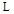of the electron when immersed within a hyperdense hadronic medium are characterized by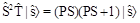(7)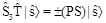(8)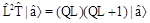(9)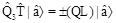(10)

where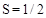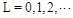, where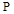and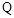are arbitrary (non-null) positive parameters and isotopically liftedandare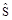and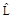respectively.

Santilli introduced the above isotopy of SU(2)-spin to prevent the belief of the perpetual motion that is inherent when the applicability of quantum mechanics is extended in the core of a star.

In fact, quantum mechanics predicts that an electron moves in the core of a star with an angular momentum that is conserved in exactly the same manner as when the same electron orbits around proton in vacuum, thus an electron in the core of a star can only have a locally varying angular momentum and spin as represented by Eqs. 7 - 10.

In case of the isoelectron in the deuteron, we have the constraint that the orbital angular momentum must be equal but opposite to that of the spin: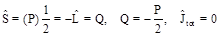(11)

The exact and invariant representation of the spin 1 of the ground state of the deuteron then follows according to the rule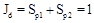(12)

Now suppose that the quantum mechanical angular momentum operatorhas expectation value 1, then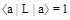(13)

Under isotopic lifting the above expression easily acquires the value 1/2 for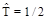,=2.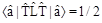(14)

However, in this case the isounit is given by. Therefore, when the isoeigenvalue of the angular momentum is properly represented as an isonumber (an ordinary number multiplied by the isounit), one recovers the original value 1.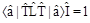(15)

thus recovering causality and other laws.

It should be noted that there is no violation of Pauli’s exclusion principle in this case since that principle only applies to "identical" particles and does not apply to protons and neutrons, as well known (more explicitly, one of the two protons of Eq. 4 is in actuality the neutron since it has embedded in its interior, the isoelectron).

4.6. Magnetic Moment of Deuteron

The experimental values of magnetic moment of deuteron and its constituents are: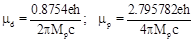(16)

and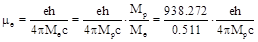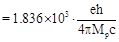(17)

We know that deuteron is in its ground state with null angular momentum and there is no orbital contribution to the total magnetic moment from the two protons. Thus the exact and invariant representation of the total magnetic moment of the deuteron is then given by: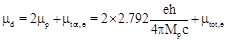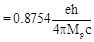(18)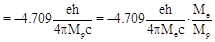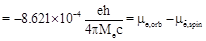(19)

In the above equation, missing contribution is provided by the total magnetic moment of the isoelectron. The latter numerical value is given by the difference between the orbital and the intrinsic magnetic moment that is very small (per electron’s standard) since the total angular momentum of the isoelectron is indeed small. Also note the correct value of the sign because the isoelectron has the orbital motion in the direction of the proton spin. But the charge is of opposite sign.

Thus the direction of the orbital magnetic moment of the isoelectron is opposite to that of the proton, as represented in equation 4. The small value of the total magnetic moment of the isoelectron for the case of the deuteron is close to the corresponding value for the neutron.

4.7. Deuteron Force

The assumption that the deuteron is a bound state of a proton and a neutron does not provide any explanation for physical origin of the nuclear forces. Quantum mechanics provides mathematical description of the attractive force via number of potentials, although none of them admits a clear physical explanation of the strong attraction between protons and neutrons. Santilli has always tried to generalize quantum mechanics for nuclear physics by providing fundamentally different notions and representations by using hadronic mechanics principles.

We have seen that Model represented by equation 4 permits a clear resolution of this additional insufficiency of quantum mechanics via the precise identification of two types of nuclear forces, the first derivable from a Coulomb potential and the second of contact type represented with the isounit. On the inspection of Figure 4 we see that the constituents of deuteron are in specific configuration such that there we have short range pair-wise opposite signs of charges and magnetic moments with long range identical signs of charges and magnetic moments. Thus it implies that the net attractive Coulomb force in the deuteron is determined by the following expression of potential: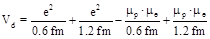(20)

In addition, the constituents admit an attractive force not derivable from a potential due to the deep penetration of their wavepackets in singlet pair-wise couplings, which force is the same as that of the two identical electrons in the Cooper and valence pairs, the structure of mesons, the structure of the neutron, and can be represented via the isounit: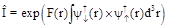(21)

The projection of the above force chracterizes a strongly attractive Hulthen potential, that behaves at short distances like the Coulomb potential, thereby absorbing the latter and resulting in a single, dominating, attractive Hulthen well with great simplification of the calculations. Thus it can be seen that besides the above potential and contact force, no additional nuclear force is needed for an exact and invariant representation of the remaining characteristics of the deuteron, such as binding and total energies. It can be proved that the isoelectron is not restricted to exist within one of the two protons, because there lies a 50% isoprobability of moving from the interior of one proton to that of the other proton. Therefore, the proton-neutron exchange is confirmed by model given by equation 4.

4.8. Deuteron Binding Energy

We know that quantum mechanics is a purely Hamiltonian theory in the sense that the sole admitted forcers are those derivable from a potential. So direct and immediate consequence is the impossibility of quantitative representation of the deuteron binding energy. The the experimental binding energy of deuteron is(22)

that is, a representation via equations, rather than via the existing epistemological arguments. Thus the mathematics underlying quantum mechanics, being local differential, can only represent the proton and the neutron of model as being point-like particles. As a result of this fact quantum mechanics admits no binding energy at all for the Deuteron, including the absence of binding energy of Coulomb type, because the neutron is abstracted as a neutral massive point. The lack of a quantum mechanical binding energy for the Deuteron persists even under the assumption that the Deuteron is composed of six hypothetical quarks because attractive and repulsive contributions between the hypothetical quarks of the proton and those of the neutron cancel out, resulting in no force acting at all between the proton and the neutron, irrespective of whether attractive or repulsive.

Model given by equation 4, under the covering laws of hadronic mechanics has permitted the achievement of the first quantitative representation of the binding as well as the total energy of the Deuteron in scientific history, thus illustrating the validity of Santilli’s original proposal of 1978  to build the covering hadronic mechanics.

According to hadronic mechanics, the binding energy is mainly characterized by forces derivable from a potential since the contact forces due to mutual wave-overlapping of wave packets have no potential energy. Hence, the binding energy of the deuteron is due to the potential component of the deuteron binding force given by equation 20. This can be verified by using known values of charges and magnetic moments for the two electron-proton pairs of the deuteron and their mutual distances.

Now, Hadronic mechanics also permits the exact and invariant representation of the total energy of the deuteron, that is direct verification of model given by equation 4.

Now 1 amu = 941.49432 MeV gives,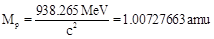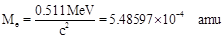The mass of a nucleus with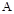nucleons and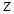protons without the peripheral atomic electrons is characterized by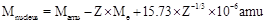(23)

and thus for deuteron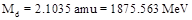(24)

The iso-Schrödinger equation for model given by equation 4 can be reduced to that of the neutron, under the assumption that the isoelectron spends 50% of the time within one proton and 50% within the other, thus reducing model (equation 4) in first approximation to a two-body system of two identical particles with un-isorenormalized mass given by(25)

The main differences are given by different numerical values for the energy, meanlife and charge radius. Thus Santilli derived the structured equation of the deuteron as a two-body nonrelativistic approximation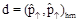(26)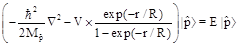(27)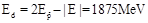(28)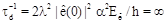(29)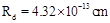(30)

The above equations admit a consistent solution reducible to the algebraic expressions as for the case of Rutherford-Santilli neutron,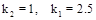(31)

It is worth noting that, in the above model, the deuteron binding energy is zero,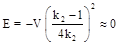(32)

because all potential contributions have been included in the structure of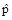and, for the binding of the twoall potential forces have been absorbed by the nonlocal forces and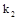has now reached the limit value of 1 (while being close to but bigger than 1). It has been observed that a more accurate description can be obtained via the restricted three-body configuration of Figure 4. This model gives an exact solution. The model can be constructed via a nonunitary transform of the conventional restricted three-body Schrödinger equation for two protons with parallel spin 1/2 and one isoelectron with null total angular momentum as per Figure 4 with conventional Hamiltonian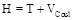, whereis given by equation 20. The nonunitary transforms then produces an additional strong Hulthèn potential that can absorb the Coulomb potential resulting in a solvable equation.

4.9. Electric Dipole Moment and Parity of Deuteron

It is well known that the electric dipole moment of the proton, neutron and Deuteron are null. The preservation of these values by hadronic mechanics is assured by the general property that axiom-preserving lifting preserves the original numerical values, and the same holds for parity. The positive parity of the deuteron is represented by hadronic mechanics via the expression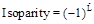(33)

The value for unperturbed deuteron in its ground state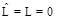. It should be noted that on one hand, the parity of the deuteron is positive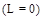, while on the other hand, in order to attempt a recombination of deuteron magnetic moments and spin, the unperturbed deuteron is assumed as being a mixture of different levels, some of which have non-null values of, thus implying the impossibility of a positive parity.

Thus Santilli has shown that the isotopic branch of nonrelativistic hadronic mechanics permits the exact and invariant representation of "all" the characteristics of the deuteron composed of two isoprotons and one isoelectron, at the same time resolving all quantum insufficiencies spelled out in the main text above.

4.10. Reduction of Matter to Isoproton and Isoelectrons

It is evident that, following the reduction of the neutron to a proton and an electron and the reduction of the deuteron to two protons and one electron, Santilli has indeed achieved the important reduction of all matter to protons and electrons, since the reduction of the remaining nuclei to protons and electron is consequential, e.g., as a hadronic bound state of two mutated deuterons represents Helium nucleus.

We would like to close our discussion by indicating Santilli’s additional astro- physical contribution given by the fact that the so-called "neutron stars" are in reality an extremely high density and high temperature fluid composed by the original constituents of the star, protons and electrons in their isoprotons and isoelectrons realization, in conditions of deep mutual penetration under the laws of hadronic mechanics.

5. Conclusion

As it is well known, the local-differential structure of quantum mechanics solely permits the representation of p[articles as being massive points. This abstraction has been proved to be effective for the representation of the structure of atoms, since the atomic constituents are at very large mutual distances compared to the size of charge distributions or wave packets of particles.

As shown by R. M. santilli in mathematical and physical details, the insufficiency of quantum mechanics to represent the characteristics of the neutron in its synthesis from the hydrogen atom in the core of a star are due precisely to the insufficiency of the representation of the proton and electron as massive points.

In fact, the representation of the proton as an extended charge distribution of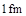radius has permitted the representation of all characteristics of the neutron as a compressed hydrogen atom in the core of stars . As an illustration, the anomalous magnetic moments of the neutron is readily represented by a contribution which is impossible for quantum mechanics, but intrinsic in the very conception of hadronic mechanics, namely, the contribution from the orbital motion of the electron when totally compressed inside the proton.

The same advances have shown that the characteristics of the electron change in the transition from isolated conditions in vacuum to the condition of total penetration within the hyperdense proton.

This difference has been quantitatively and invariantly represented by Santilli via, firstly, the transition from Lie’ theory to the covering lie-Santilli isotheory, and, secondly, via the transition from particles to isoparticles, namely, the transition from irreducible unitary representations from the conventional Lorentz symmetry to those of the covering Lorentz-Santilli isosymmetry. An exact and time invariant representation of all characteristic of the neutron as a generalized bound state of one isoproton and one isoelectron then follow.

Following, and only following the achievement of a constant, exact and invariant representation of the structure of the neutron Santilli has applied the results to the structure of the deuteron conceived as a three-body generalized bound state of two isoprotons and one isoelectron .

This has permitted the exact and invariant representation of all characteristics of the deuteron, with intriguing implications, such as the reduction of all matter in the universe, to protons and electrons in various dynamical conditions.

As an illustration, Santilli’s astrophysical contributions finds their root in the fact that the so-called "neutron stars" are in reality an extremely high density and high temperature fluid composed by the original constituents of the star, protons and electrons, in conditions of deep mutual penetration under the laws of hadronic mechanics.

Needless to say, a virtually endless list of intriguing open problems have emerged from the above new vistas in nuclear physics,m among which we mention: the need to reexamine from its foundation the notion of nuclear force due to the emergence of a component not derivable from a potential whose control may lead to new clean nuclear energies; the implications of Santilli’s deuteron structure on the natural radioactivity elsewhere; the exact and invariant representation of the spin and magnetic moments of all nuclei; and others.

Acknowledgments

The author would like to thanks Prof. R. M. Santilli and Prof., A. A. Bhalekar for invaluable assistance in the preparation of this paper.

References

1. R. M. Santilli, J. New Energy, Vol. 1, 1, (1999). http://www.santilli-foundation.org/docs/Santilli-114.pdf
2. R. M. Santilli, Intern. J. Phys., Vol. 4, 1, (1998). http://www.santilli-foundation.org/docs/Santilli-07.pdf
3. R. M. Santilli, "A quantitative isotopic representation of the deuteron magnetic moment," in Proceedings of the International Symposium, "DUBNA DEUTERON-93", Joint Institute for Nuclear Research, Dubna, Russia (1994) http://www.santilli-foundation.org/docs/Santilli-134.pdf
4. R. M. Santilli, "Theory of mutation of elementary particles and its application to Rauch’s experiment on the spinorial symmetry," ICTP preprint # IC/91/265 (1991) http://www.santilli-foundation.org/docs/Santilli-141.pdf
5. R. M. Santilli, "The notion of nonrelativistic isoparticle," ICTP preprint # IC/91/265 (1991) http://www.santilli-foundation.org/docs/Santilli-145.pdf
6. R. M. Santilli, Elements of Hadronic Mechanics, vol. I and II, Naukkova Dumka Publishers, Kiev, second edition, 1995. http://www.santilli-foundation.org/docs/santilli-300.pdfhttp://www.santilli-foundation.org/docs/santilli-301.pdf
7. R. M. Santilli, Hadronic Mathematics, Mechanics and Chemistry, Vol. I [a], II [b], III [c], IV [d] and V [e], International Academic Press, Palm Harbor, Florida, U.S.A. http://www.i-b-r.org/Hadronic-Mechanics.htm
8. I. Gandzha and J. Kadeisvily, New Sciences for a New Era. Mathematical, Physical Discoveries of Ruggero Maria Santill, Sankata Printing Press, Kathmandu, Nepal, 2011.
9. C. S. Burande, Numerical Analysis and Applied Mathematics ICNAAM 2013 AIP Conf. Proc. 1558, 693 (2013). http://dx.doi.org/10.1063/1.4825586 http://www.santilli-foundation.org/docs/burande.pdf
10. R. M. Santilli, The Structure of the neutron as predicted by Hadronic Mechanics, http://www.neutronstructure.org.
11. R. M. Santilli, The Structure of the neutron as predicted by Hadronic Mechanics, http://www.neutronstructure.org.
12. R. M. Santilli, Elements of Hadronic Mechanics, vol. I and II, Naukkova Dumka Publishers, Kiev, second edition, 1995. http://www.santilli-foundation.org/docs/santilli-300.pdfhttp://www.santilli-foundation.org/docs/santilli-301.pdf
13. V. Krasnoholovets, Scientific Inquiry, Vol. 7, no. 1, 25, June 30, (2006).
14. V. Krasnoholovets and D. Ivanovsky, "Motion of a particle and the vacuum", Physics Essays Vol. 6, no. 4, 554-263 (1993) (arXiv.org e-print archive; http://arXiv.org/abs/quant-ph/9910023).
15. V. Krasnoholovets, "Motion of a relativistic particle and the vacuum". Physics Essays Vol. 10, no. 3, 407-416 (1997) (arXiv.org e-print archive quant-ph/9903077).
16. V. Krasnoholovets, "On the nature of spin, inertia and gravity of a moving canonical particle". Indian J. Theor. Phys., Vol. 48, no. 2, 97-132 (2000b) (also arXiv.org e-print archive; http://arXiv.org/abs/quantph/ 0103110).
17. L. Hulthén, Ark. Mat. Astron. Fys., 28A, 5 (1942).
18. R. M. Santilli, Hadronic J. Vol. 1, 574-901 (1978). http://www.santilli-foundation.org/docs/santilli-73.pdf

i This work was partly presented at the International Conference of Numerical Analysis and Applied Mathematics (ICNAAM) - 2014, at Rhodes, Greece, September 22-27, 2014.

 Contents 1. 2. 3. 3.1. 3.2. 4. 4.1. 4.2. 4.3. 4.4. 4.5. 4.6. 4.7. 4.8. 4.9. 4.10. 5.
Article ToolsAbstractPDF(2421K)